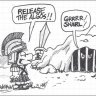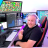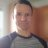# New High vs. New Low Index / Leading Indicator by Mobius for ThinkorSwim#### markos

##### Well-known member
VIP
The Chart below is of the Market Meltdown in January, 2016. It's hard to be Bearish on the Market as a whole when new highs are breaking out! This is a leading indicator.

https://tos.mx/VhAvabCode:
``````#This indicator uses the Index New highs and Index New lows
#to help gauge overall Market sentiment. It's a leading indicator.
# Index New High - New Low Indicator
# Mobius v2018-March from JQ's OneNote

declare lower;
input Symb = {default "NYSE", "NASDQ", "AMEX", "ARCA", "ETF"};
input length = 10;
input OverSold = 20;
input OverBought = 80;
Input AvgType = AverageType.Hull;

def agg = AggregationPeriod.Day;
def NYSEH  = close(Symbol = "\$NYHGH", period = agg);
def NYSEL  = close(Symbol = "\$NYLOW", period = agg);
def NASDQH = close(Symbol = "\$NAHGH", period = agg);
def NASDQL = close(Symbol = "\$NALOW", period = agg);
def AMEXH  = close(Symbol = "\$AMHGH", period = agg);
def AMEXL  = close(Symbol = "\$AMLOW", period = agg);
def ARCAH  = close(Symbol = "\$ARHGH", period = agg);
def ARCAL  = close(Symbol = "\$ARLOW", period = agg);
def ETFH   = close(Symbol = "\$ETFHGH", period = agg);
def ETFL   = close(Symbol = "\$ETFLOW", period = agg);
def P;
Switch (Symb){
case "NYSE":
P = NYSEH / (NYSEH + NYSEL) * 100;
case "NASDQ":
P = NASDQH / (NASDQH + NASDQL) * 100;
case "AMEX":
P = AMEXH / (AMEXH + AMEXL) * 100;
case "ARCA":
P = ARCAH / (ARCAH + ARCAL) * 100;
case "ETF":
P = ETFH / (ETFH + ETFL) * 100;
}
def price = if isNaN(P) then price else P;
plot data = if isNaN(close) then double.nan else price;
data.EnableApproximation();
data.SetDefaultColor(Color.Cyan);
plot avg = MovingAverage(AvgType, data, length);
avg.EnableApproximation();
avg.AssignValueColor(if between(avg, OverSold, OverBought)
then Color.yellow
else if avg >= OverBought
then Color.Green
else Color.Red);
avg.SetLineWeight(2);

plot OB = if isNaN(close) then double.nan else OverBought;
OB.SetDefaultColor(Color.Red);

plot OS = if isNaN(close) then double.nan else OverSold;
OS.SetDefaultColor(Color.Green);

plot neutral = if isNaN(close) then double.nan else 50;
neutral.SetdefaultColor(Color.Dark_Gray);

# End High - Low Index``````

Last edited by a moderator:
•Sport87 and mc01439
G

#### gamgoum

##### New member
Hello and thanks for sharing, I was looking around for a US total new High - New low indicator but could not find it on think or swim, my best approximation was to add up these TOS indicator in a rudimentary formula:
\$AMHI1M+\$ARHI1M+\$NAHI1M+\$NYHI1M+\$ETFHI1M-\$AMLO1M-\$ARLO1M-\$NALO1M-\$NYLO1M-\$ETFLO1M
Which can be plotted in TOS but is not still represnting the full US new High - New lows

I would appreciate any leads on that

GamSet Position Exit to Low/High before Entry Bar? Questions 2Need to create an audible alert for new 10-minute HIGH/LOW... Questions 5Near or at High/Low of Day/Year question??? Questions 4